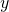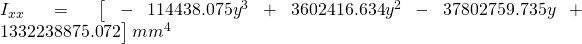# Finding an equation for the Moment of Inertia of a complex tapering member using Python

Often in engineering you need to make simplification in analyses that make your life easier, often applying some judgement based on experience that involves some simplification in the analysis of a structure for example. Sometimes you do this in the name of achieving conservatism, other times to get a reasonable/faster answer that is deemed close enough to the true solution to be accepted as good enough.

Sometimes peoples understanding of engineering principles lets them down here, and the end result is they made it simple, but they also made it wrong or unconservative. Don’t be this person.

One such case of simplification is when you are dealing with tapering members. Often when modelling these members in any analysis package you’ll discretise the member into a series of shorter elements with constant section/material properties over each sub-segment. These approximate properties are based on the properties at the midpoint or one end of the subdivided section.

Take for example a tapered pole, both the Moment of Inertia and the lateral wind loading on it might change with height above the base for example.

Using a first principles approach if you knew the equation for the load in terms of a heightabove the base and an equation for the Moment of Inertia in terms of a heightabove the base then you could calculate the exact deflection using any hand method.

Now in practice you wouldn’t do this by hand, you’d just make some simplification and move on and accept that if you subdivided the member into short enough segments that the answer would be within some reasonable margin of the true result. But its a good example of using python to find this equation, so we’re diving in anyway!

To do this, as noted you’re going to need an equation that describes the Moment Of Inertia along the member. Now working out an equation based on the actual section parameters like the thickness of walls, width/depth of the section can be done for relatively simple shapes where the change in geometry is linear with height. However if you have something complex like a highly complex shape that has a thickness/width/depth that varies non linearly with height then this will become hard real fast (basically think of any weird shape architects would like).

Never fear though, there is an easy(ish) way with a bit of Python and the section-properties package. If we can evaluate the section properties required at a reasonable amount of stations along our member, and knowing the changes in geometry at these stations, we can then use some curve fitting techniques to derive an equation for how the properties we are interested in change along the length of the member. We could then use an equation derived for the Moment of Inertia to evaluate the deflection for example (perhaps the subject of a future post to compare the true calculated deflection vs one calculated in an analysis package using segments with constant properties, provided I can remember how to do this from first principles!).

Similar to this post, to evaluate the section properties I am using the excellent section-properties package to do the heavy lifting for calculating the section properties at a limited number of discrete locations. Then using some curve fitting via the numpy polyfit function we can derive our equation. This is followed by some testing of this equation at closer spaced stations to show perfect agreement.

The section I’m considering is an imaginary 8 meter long tapered pole like you might see for a streetlight or similar, made from thin cold formed steel. Considering a 10 sided polygon with 6mm plate with a 5mm internal radii, with a PCD of 400mm at the base and 100mm at the top and the PCD changing linearly with height (i.e. a constant taper).

Lets get onto the results:-

As we can see the equation for this particular case is:-The python code to achieve this is as follows:-

import matplotlib.pyplot as plt
import numpy as np
import sectionproperties.pre.sections as sections
from sectionproperties.analysis.cross_section import CrossSection
from sympy import S, printing, symbols

# height steps
ht = 8  # m

# number of initial analysis points
steps = ht + 1  # every 1.0m

# factor for increasing the number of steps for testing derived equation
refined_steps_factor = 5

# Wall thickness
t=6

# top and bottom PCD's
top_d = 100
bot_d = 400

# create height steps array
ht_array = np.linspace(0, ht, num=steps)

# create diameter steps array
dia_array = np.linspace(bot_d, top_d, num=steps)

# create empty results array
I_results = np.empty(steps)

# evaluate at initial points to form basis for curve fitting
for i in range(len(ht_array)):
geometry = sections.PolygonSection(d=dia_array[i], t=t, n_sides=10, r_in=5, n_r=12)

mesh = geometry.create_mesh(mesh_sizes=)

#geometry.plot_geometry()
section = CrossSection(geometry, mesh)
#section.plot_mesh()
print('Evaluating diameter ' + str(dia_array[i]))
section.calculate_geometric_properties()
ixx_g, iyy_g, ixy_g = section.get_ig()
print('Ixx = ' + str(ixx_g))

I_results[i] = ixx_g

# create height steps array
ht_array1 = np.linspace(0, ht, num=steps * refined_steps_factor)

# create diameter steps array
dia_array1 = np.linspace(bot_d, top_d, num=steps * refined_steps_factor)

# create empty results array
I_results1 = np.empty(steps * refined_steps_factor)

# evaluate at closer ctrs to compare with equation
for i in range(len(ht_array1)):
geometry = sections.PolygonSection(d=dia_array1[i], t=t, n_sides=10, r_in=5, n_r=12)

mesh = geometry.create_mesh(mesh_sizes=)

# geometry.plot_geometry()
section = CrossSection(geometry, mesh)
# section.plot_mesh()
print('Evaluating diameter ' + str(dia_array1[i]))
section.calculate_geometric_properties()
ixx_g, iyy_g, ixy_g = section.get_ig()
print('Ixx = ' + str(ixx_g))

I_results1[i] = ixx_g

# calculate polynomial fit (cubic curve)
z = np.polyfit(ht_array, I_results, 3)
# print Polynomial coefficients, highest power first
print(z)
f = np.poly1d(z)

# setup equation text for legend
y = symbols('y')
poly = sum(S("{:6.3f}".format(v)) * y ** i for i, v in enumerate(z[::-1]))
eq_latex = 'Ixx = ' + printing.latex(poly)

# calculate new x's and y's for testing eqn
ht_new = np.linspace(ht_array, ht_array[-1], steps * refined_steps_factor)
I_new = f(ht_new)

# plot the moment of inertia as a function of height
# initialise plot
(_, ax) = plt.subplots()

ax.plot(I_results, ht_array, 'ko', ms=8, label='INITIAL POINTS FOR CURVE FITTING')

ax.plot(I_results1, ht_array1, 'ro--', lw=1, ms=4, label="".format(eq_latex))

ax.plot(I_new, ht_new, 'kx', ms=8, label='CURVE FITTING VALIDATION POINTS')

plt.legend(fontsize='small')

ax.set_xlabel('MOMENT OF INERTIA (Ixx)')
ax.set_ylabel('HEIGHT ABOVE GROUND (y)')
ax.set_title('MOMENT OF INERTIA vs HEIGHT')

plt.show()


The beauty of this numerical technique is no matter how complex the transition in geometry is you can create a single equation for the Moment of Inertia in terms ofthe height above the base. Similarly because you have the coefficients of the polynomial they can be used directly in Python for any code you might create that follows.

The same concept would apply for calculating any other property, like for example the plastic section modulus could be calculated in this manner to give an equation for the sections bending capacity along the member. This capacity could be compared directly to the moment demand (if there were no lateral torsional buckling issues obviously)!

As another example you could consider a welded I-section made up from plate, you could transition the top and bottom flange widths independently and also the depth of the web.